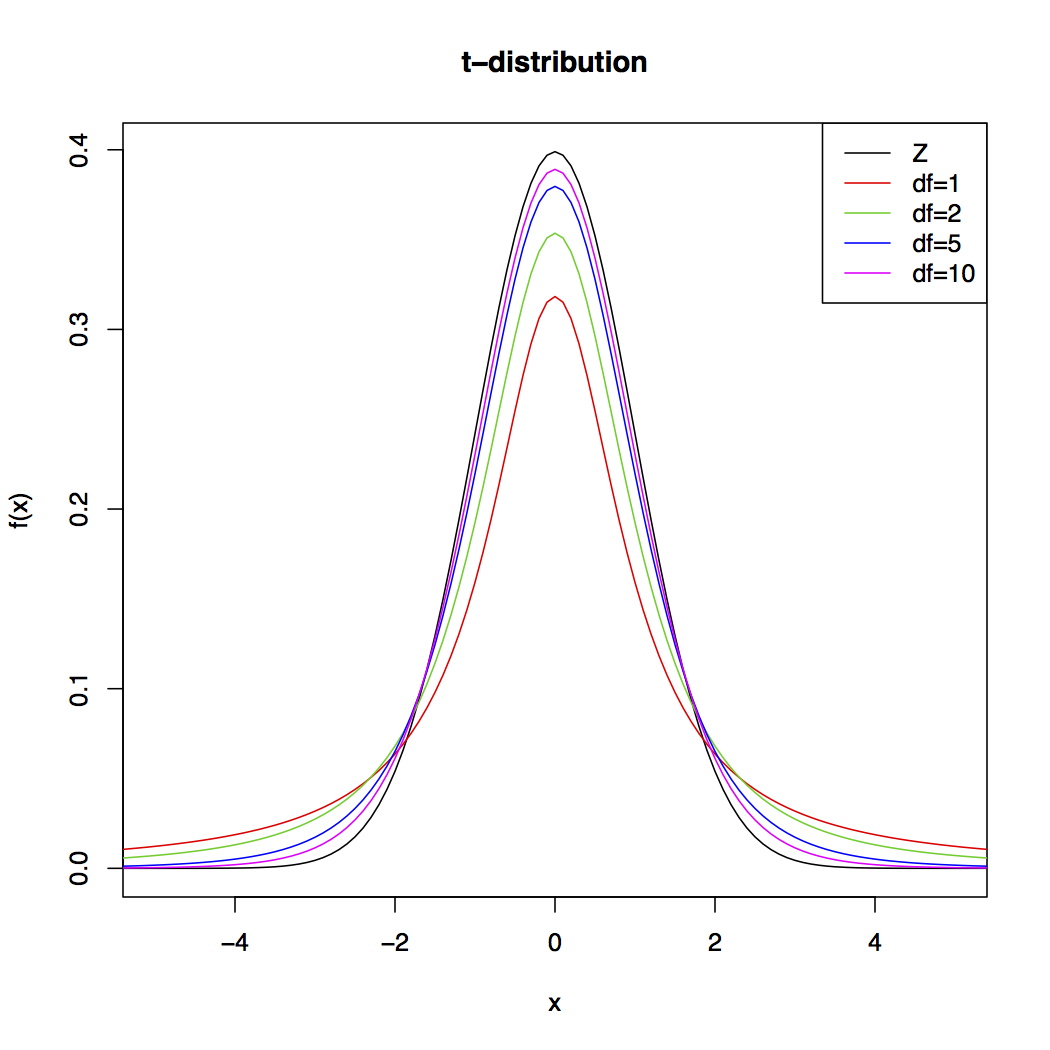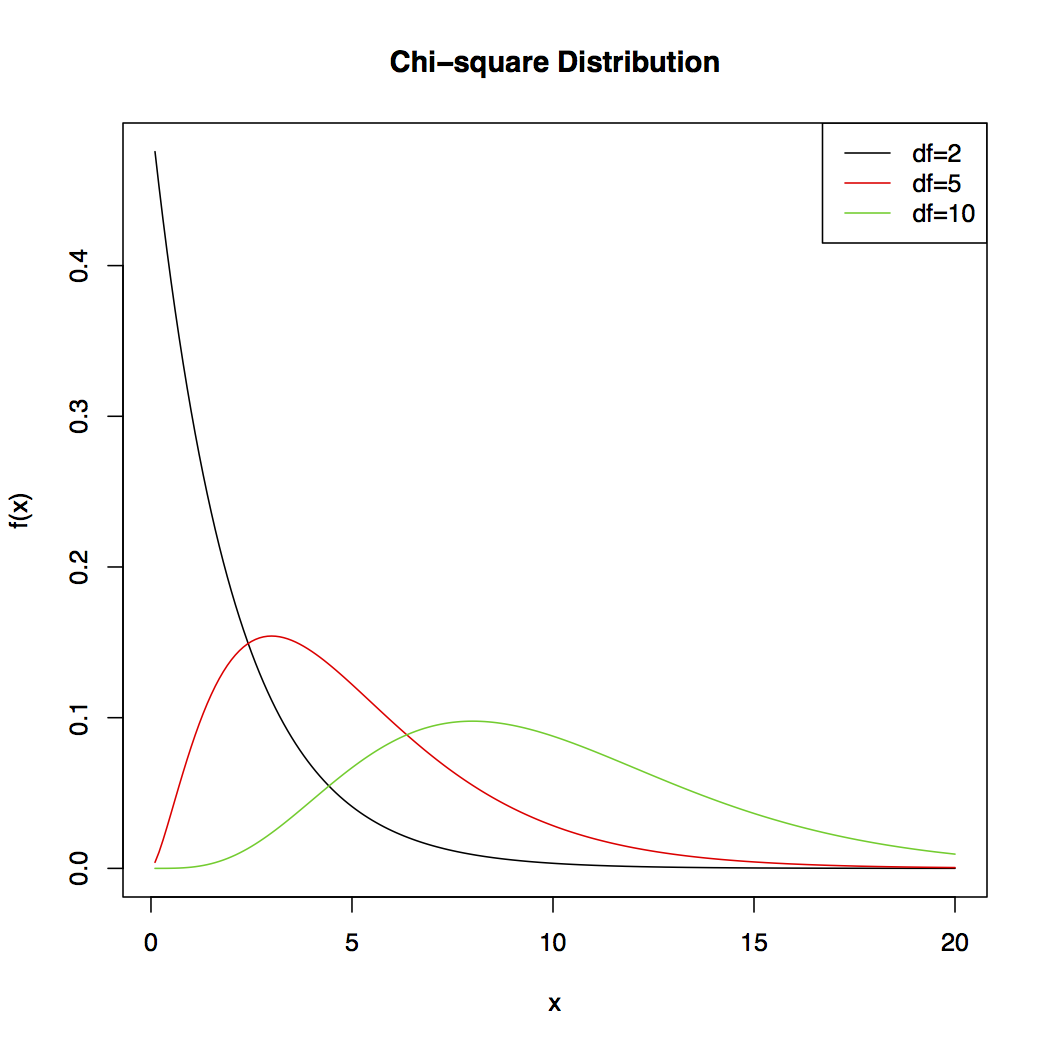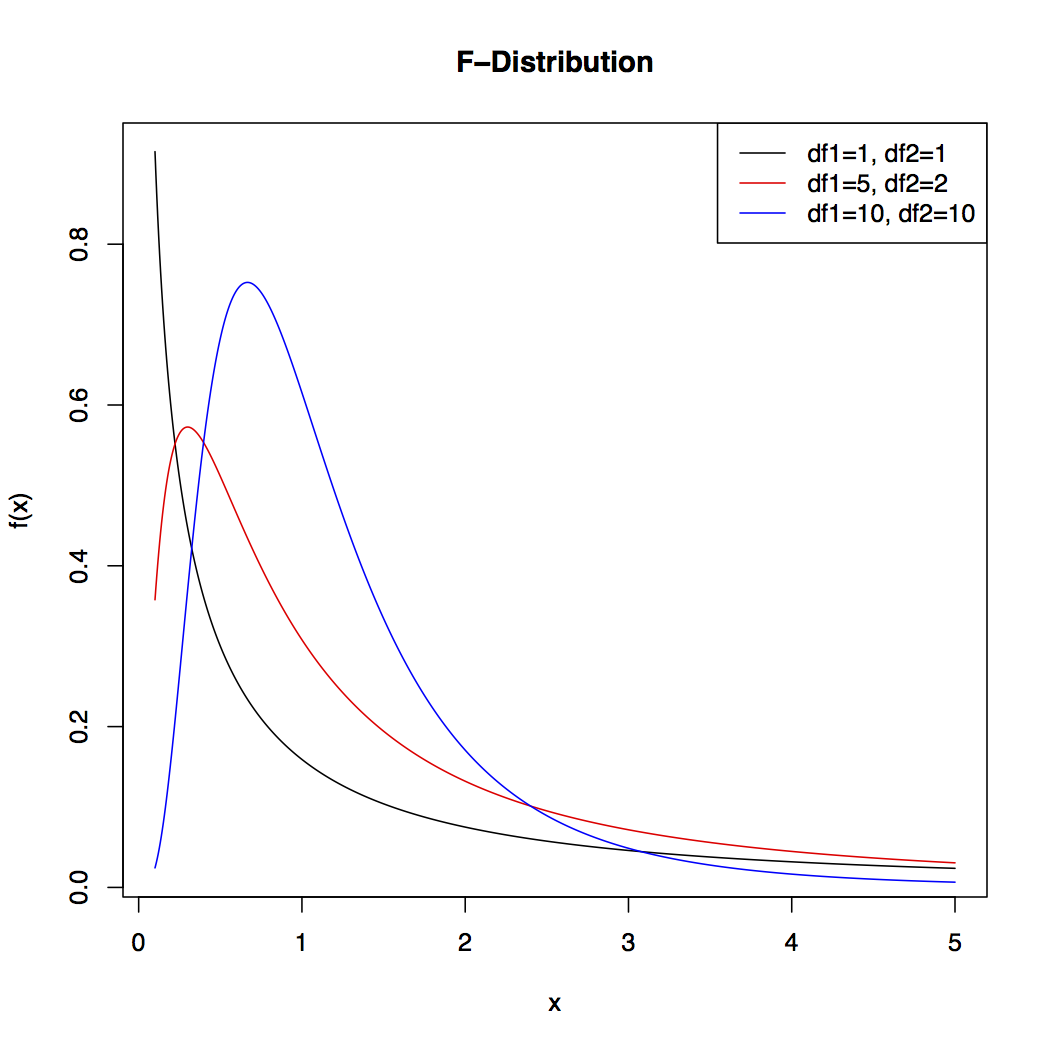# 3.3.5 - Other Continuous Distributions

3.3.5 - Other Continuous Distributions

Although the normal distribution is important, there are other important distributions of continuous random variables. Some we will introduce throughout the course, but there are many others not discussed. Here are a few distributions that we will see in more detail later.

The t-distribution is a bell-shaped distribution, similar to the normal distribution, but with heavier tails. It is symmetric and centered around zero. The distribution changes based on a parameter called the degrees of freedom. We will discuss degrees of freedom in more detail later.

The graph shows the t-distribution with various degrees of freedom. The standard normal distribution is also shown to give you an idea of how the t-distribution compares to the normal. As you can see, the higher the degrees of freedom, the closer the t-distribution is to the standard normal distribution.The chi-square distribution is a right-skewed distribution. The distribution depends on the parameter degrees of freedom, similar to the t-distribution. Here is a plot of the Chi-square distribution for various degrees of freedom.

We will see the Chi-square later on in the semester and see how it relates to the Normal distribution.The F-distribution is a right-skewed distribution. The distribution depends on the two parameters both are referred to as degrees of freedom. The first is typically called the numerator degrees of freedom ($d_1$) and the second is typically referred to as the denominator degrees of freedom ($d_2$). Here is a plot of the F-distribution with various degrees of freedom.

The F-distribution will be discussed in more detail in a future lesson.Link ↥ Has Tooltip/Popover Toggleable Visibility